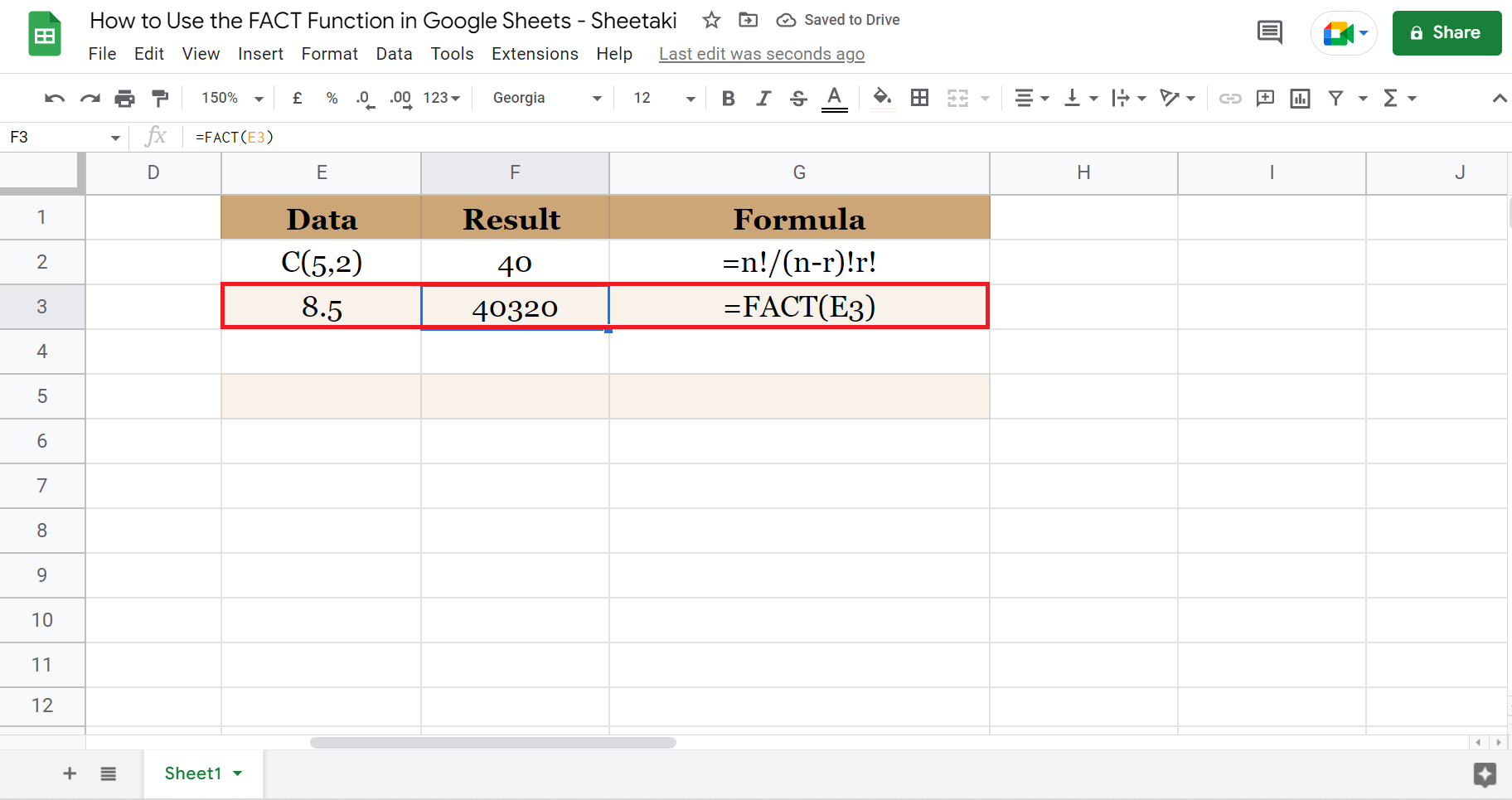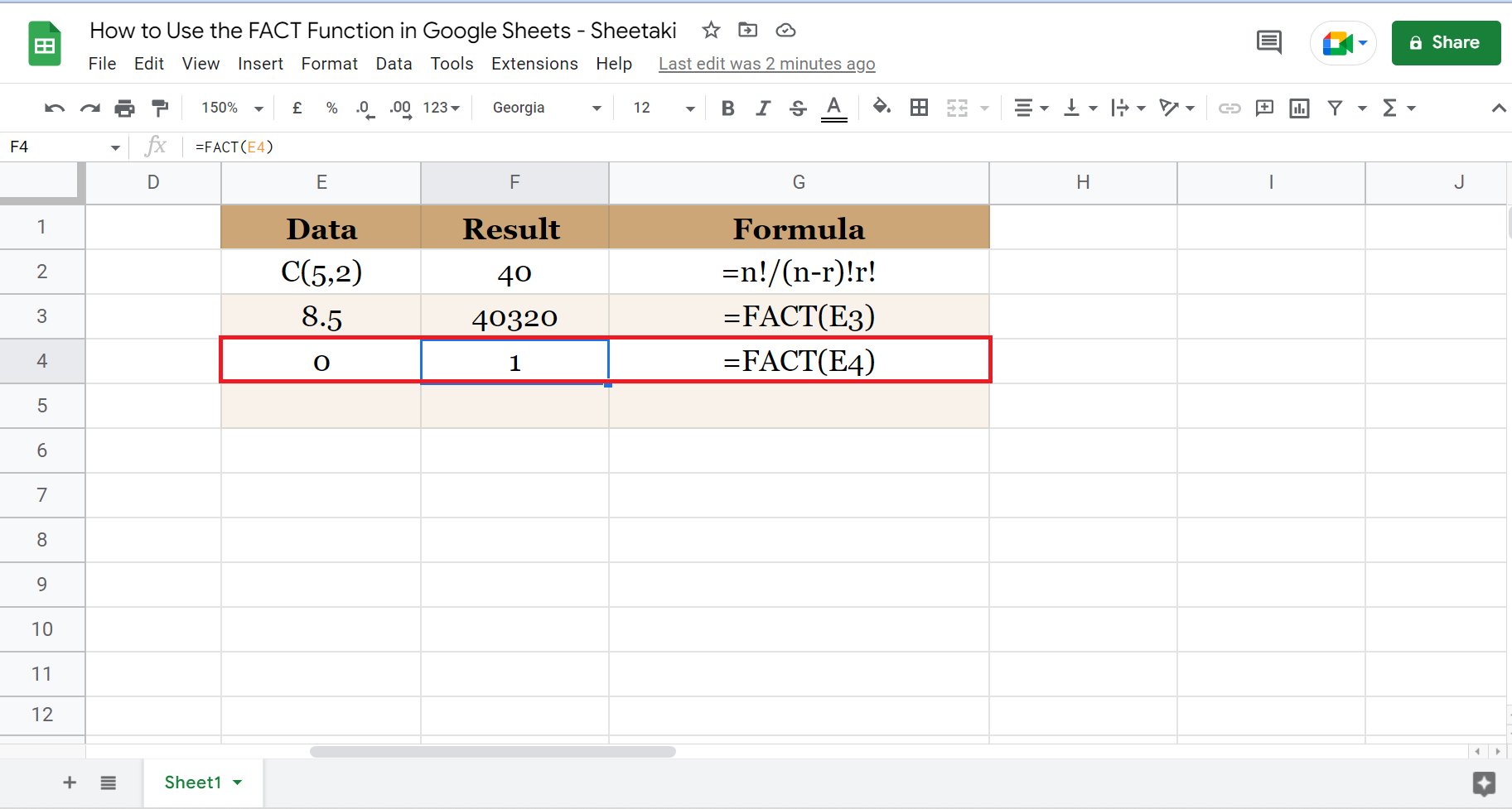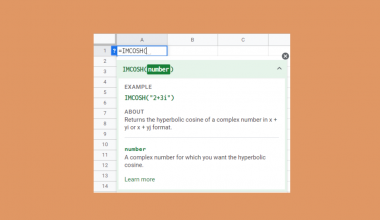# How to Use the FACT Function in Google Sheets

This guide will discuss how to use the FACT function in Google Sheets to get the factorial of a number.

Instead of manually multiplying all the integers to get the factorial, we can simply use the `FACT` function to do the calculations and return the factorial of a number.

The rules for using the `FACT` function in Google Sheets are the following:

• The number used to calculate the factorial should be greater than or equal to 0.
• The number used to calculate the factorial should be less than or equal to 170.

A factorial is the product of a number and all the positive integers less than or equal to that number. Also, factorials are used in permutation and combination.

And a permutation is used to calculate the number of all the possible arrangements of a set if the order of the arrangement matters.

Otherwise, a combination is used to calculate all the selections of a set if the order of the arrangement does not matter. So the permutation or the combination of a set is represented by the factorial.

Let’s take a scenario where you need to use the `FACT` function in Google Sheets.

Suppose you are planning a dinner party for the company. And all the employees of each department will be present. So you want to determine all the possible seating arrangements for a table of 10 would be during the event.

In other words, you need to calculate the permutation of seating arrangements for a table of 10. To do this efficiently, you use the `FACT` function in Google Sheets to get the answer. Hence, you determined that you have 3,638,800 ways to arrange the seating arrangements.

Simple and efficient in getting the factorial! Let’s move on and learn how we write the `FACT` function in Google Sheets.

## The Anatomy of the FACT Function

The syntax or the way we write the `FACT` function is as follows:

` =FACT(value)`

Let’s take each term and try to understand what they mean:

• = this is the equal sign. It is how we always start any function in Google Sheets.
• FACT() this is our `FACT` function. This function takes any number or cell containing the number you want to use and calculates the factorial.
• value refers to the number you want to calculate the factorial of. This value must be greater than or equal to 0. Otherwise, Google Sheets will return an error. Similarly, Google Sheets will return it as an error if the value is greater than 170. Since the factorial will be too large for Google Sheets to represent.

Note: If the value is in decimal, Google Sheets will simply refer to the whole number and return the factorial of that number.

## A Real Example of Using the FACT Function in Google Sheets

Now that we have learned the syntax of the `FACT` function. Let’s examine a real example of using the `FACT` function in Google Sheets. For instance, we want to get the factorial of the numbers 11-20. First, check the spreadsheet below.To get the result, we simply need to input the `FACT` function and select the cell that contains the number we will calculate the factorial of. After, this is what it would look like.Another example of using the `FACT` function in Google Sheets is for calculating combinations. Let’s say we want to determine how many groups we can make from a set of 5 if we take 2 each time.A combination is written as C(n, r). So n is the total number of items in the set, while r refers to the number of items selected from the set. So the formula for getting a combination is `=n!/(n-r)!r!`. And the exclamation point refers to a factorial.

Great! We have examined examples of using the `FACT` function in Google Sheets. It is now time to learn the step-by-step process of how to do it.

## How to Use the FACT Function in Google Sheets

This section will explain the steps in using the `FACT` function in Google Sheets.

1. First, you can select any cell to make it active. I prepared a table to show the data, results, and formulas easily. In this example, let’s determine the factorial of the numbers 11-20 using the `FACT` function.2. Second, type in ‘=’ the equal sign to start the function. Then, type in ‘FACT’ or select it from the dropdown menu that will appear.3. Third, select the cell that contains the value or number you want to calculate the factorial of. In this case, select A2. Then, press enter to return the factorial.4. Next, drag down the formula to copy or simply accept the autofill to apply the formula to the rest of the rows.5. Finally, you have calculated the factorials for all the data using the `FACT` function. Also, take note of the formulas on the right side for your reference.6. Additionally, let’s try to calculate a combination using the `FACT` function. For instance, we want to find the combination of 2 from a set of 5. So this would be written as C(5,2).7. Then, the formula for getting a combination is n!/(n-r)!r!. Since n refers to the total number of items in the set, n is 5. While r would be 2.8. Using the `FACT` function, the formula we will use in Google Sheets is ‘=FACT(5)/FACT(5-2)*2’. And this would return a result of 40 combinations.9. If we want to find the factorial of a decimal, it is possible. For instance, let’s take 8.5 and follow the same procedure. First, type in ‘=’ the equal sign and select ‘FACT’ from the dropdown menu that appears when you type in the function’s name. Then, select the cell which is E3. Lastly, press enter to return the result.10. Furthermore, it is also possible to calculate the factorial of 0. Again, follow the same steps as before. Type in the `FACT` function and select the cell, which is E4. Finally, press enter to return the result.11. But, we cannot calculate the factorial of any number less than 0. Google Sheets will return an error of #NUM! along with a reminder that the value should be positive or equal to 0.12. Lastly, Google Sheets cannot calculate the factorial of a number that is too large. Specifically, the value cannot be greater than 170 since Google Sheets cannot represent a large factorial. If you try, it will return as an error and will remind you that the value should be less than or equal to 170.And that’s it! It was simple and easy to do, right? Now you can save time manually multiplying all the integers of a number. Instead, you can use the `FACT` function in Google Sheets to calculate the factorial of a number and even permutation and combination.

Are you interested in learning more about what Google Sheets can do? You can now use the `Fact` function in Google Sheets and the various other Google Sheets formulas available to create great worksheets that work for you. Make sure to subscribe to our newsletter to be the first to know about the latest guides and tutorials from us.Our goal this year is to create lots of rich, bite-sized tutorials for Google Sheets users like you. If you liked this one, you'll love what we are working on! Readers receive ✨ early access ✨ to new content.

##### You May Also Like## How to Restrict Data in Google Sheets with Data Validation

Learning how to restrict data in Google Sheets with data validation is useful to prevent others from entering…## How To Rotate Text In Google Sheets

Knowing how to rotate text in Google Sheets is useful if you want to change the rotation of…## How to Use IMREAL Function in Google Sheets

This guide will discuss how to use the IMREAL function in Google Sheets. When we want to extract…## How To Use KURT Function in Google Sheets

The KURT function in Google Sheets is used for computing the kurtosis of a dataset, which is one…